# Oxidation of Organic Compounds

Introduction

A common area of focus in the drinking water and wastewater is the formation of disinfection by products (DBP’s). DBP’s are formed from the oxidation of natural organic matter. Common DBP’s such as trihalomethane, haloacetic acid, and chlorite are all known to be carcinogenic. High performance liquid chromatography (HPLC) is a common detection method for the formation of DBP’s. In this experiment, hypochlorous acid (HOCl) and chloramines (NH2Cl) oxidize phenol and resorcinol into DBP’s.  By monitoring the concentrations of resorcinol and phenol, the formation of DBP’s can be analyzed.

Materials and Methods

A 200 mL phosphate buffer at pH 6.8 was split into two 100 mL solutions. The experiment was conducted in a buffered solution so that the HOCl would not impact the pH of the solution and affect the oxidation of the phenol and resorcinol.  10 mL of 2 mM phenol and resorcinol stock was added into each of the 100 mL phosphate buffers, forming a 200 μM concentration of phenol and resorcinol.  HPLC vials were prepared by adding 4 drops of thiosulfate into 8 HPLC vials; the thiosulfate was added to halt the reaction the moment the solutions were added to the vials. Lids were placed on the vials so that the thiosulfate did not equilibrate with the atmosphere. The two rows of four vials were labeled for 0,1,5, and 10 minute reaction times. 1.5 mL of each of the 100 mL phenol and resorcinol were added to the zero minute vial prior to adding HOCL or NH2Cl.  10 mL of 2 mM HOCl stock solution was added to one of the phenol and resorcinol stocks. The solution was mixed for ten minutes, 1.5 mL samples were taken at 1,5, and 10 minutes into mixing and added to the prepared HPLC vials. 2 mM NH2Cl was added to the other 100 mL phenol and resorcinol stock.  The same procedure was taken for NH2Cl samples as for the HOCl, for the remaining HPLC vials.  Both samples were then analyzed in the HPLC.

Results

Analyzing figure (1), it can be seen that Resorcinol reacts faster than Phenol.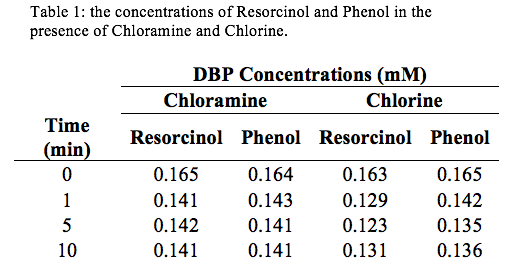Discussion:

Looking at the concentrations of Chloromine and Chlorine over a period of 10 minutes, it’s evident that the stronger oxidant is Chlorine. This is because the concentrations of Resorcinol and Phenol are lowest at t=10 minutes when in the presence of Chlorine. Using the slope-intercept equation: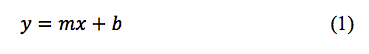We can derive an equation for concentration as a function of absorbance. This equation looks like the following: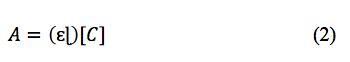Where A is absorbance,  is extinction coefficient, C is concentration,  is path length, and b (initial absorbance) is equal to 0. After manipulating equation (2) we can get an equation for concentration. From the absorbance data that we collected from the HPLC we can determine a slope ( from linear regression, then use equation (2) to derive our concentrations.  The figures for the data collected are the following: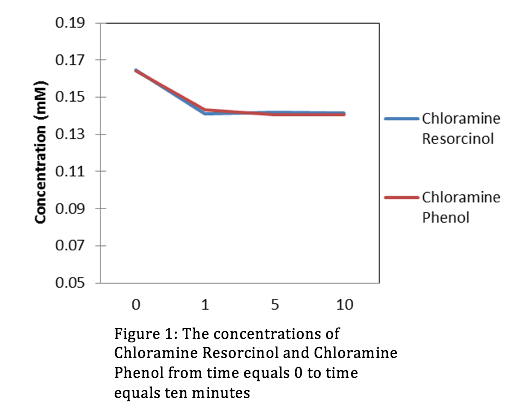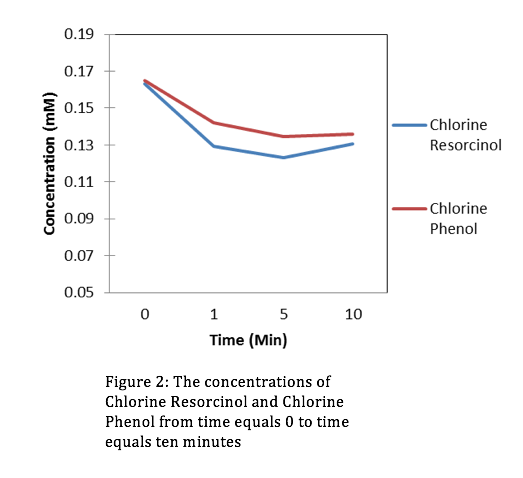Evaluation: This lab was simple and straight forward. We gained comfort with the HPLC and were introduced to organic chemistry for the first time this semester. In upcoming years don’t hesitate to add more material.

• Share